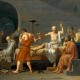Categories

# Dynamical Systems in Cosmology Lectures

In the final two lectures of my differential equations class , I discussed how Dynamical Systems theory can be used to understand and describe the dynamics of cosmological solutions to Einstein’s field equations. Videos and lecture notes posted below:

Lecture Notes:## By Dr. Ikjyot Singh Kohli

Sikh, Theoretical and Mathematical Physicist, main research in the structure and dynamics of Einstein's field equations.

## 2 replies on “Dynamical Systems in Cosmology Lectures”pablovegansays:

Cool